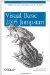# 2.5. Arrays

As in VB 6, a VB 2005 array is a collection of variables in which each variable is identified by an index, like mailboxes on a street or players on a team.

For example, the following declaration defines num1 as an array by adding open and closed parentheses to its name:

` Dim num1() As Integer `

Note that this declaration simply declares num1 to be an array; the actual size of the array is not known yet. To get num1 to point to an actual array, use the New keyword:

` num1 = New Integer() {1, 2, 3} `

num1 is now an array containing three members of Integer data type with values 1, 2, and 3.

Here are some other possible ways to declare and initialize an array:

` Dim num2(2) As Integer num2(0) = 1 num2(1) = 2 num2(2) = 3 `

The size of the array is one plus the number declared, as is the case in VB 6. In the above case, the valid index is from 0 to 2, giving a total of 3 members. Note that the following is not allowed:

` Dim num2(2) As Integer = New Integer '---Not allowed since size is '   already indicated `

You can also combine the declaration together with the initialization:

` Dim num3() As Integer = _         New Integer() {1, 2, 3} `

The following are not allowed:

` Dim num3() As Integer = New Integer() '---Not allowed; missing {} Dim num3() As Integer = New Integer(3) '---Not allowed; missing {} Dim num3() As New Integer '---Not allowed, arrays cannot use New Dim num3() as New Integer() {1,2,3} '---Syntax error `

Once an array is declared, you can change its size by using the ReDim keyword:

` Dim num4() As Integer() = New Integer() {1, 2, 3} ReDim num4(5) `VB 6 Tip: In VB 6, you can only ReDim an array if the array is initially declared as a variable length array, as the following shows: ` ' array is fixed length Dim num1(3) As Integer ReDim num1(5) '---error ' array is variable length Dim num2() As Integer ReDim num2(5) '---OK `

When an array is redimensioned, all its previous values will be lost. To retain the previous values, use the Preserve keyword:

` ReDim Preserve num4(5) `

VB 2005 adds the new To keyword. You can explicitly specify the range of an array using the To keyword:

` Dim num1(0 To 19) As Integer `Note that the To keyword is used only to make your code more readable; you cannot alter the lower bounds of the array to, say, 1. The only bound must be 0.

Note that in VB 6, you can change the base of an array using the Option Base statement. However, VB 2005 does not allow you to change the base of an array.Visual Basic 2005 Jumpstart
ISBN: 059610071X
EAN: 2147483647
Year: 2005
Pages: 86
Authors: Wei-Meng Lee

Similar book on Amazon